true
true

Mandeep K.Sector-60 Mohali, Chandigarh, India - 160059# Mandeep K.## Academic Tutor & Cuemath Teacher

Sector-60 Mohali, Chandigarh, India - 160059.

13 Students taught

5.0

UrbanPro Rating
Referral Discount: Get ₹ 500 off when you make a payment to start classes. Get started by Booking a Demo.

Details verified of Mandeep K.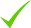IdentityEducation

Know how UrbanPro verifies Tutor details

Identity is verified based on matching the details uploaded by the Tutor with government databases.

## Overview

I am fully dedicated and passionate for my job as a teacher. TEACHING many students at my home and students as well as parents are fully satisfied with my way of teaching.

## Languages Spoken

Hindi Proficient

English Proficient

Punjabi Proficient

## Education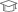Kurukshetra University 2005

Bachelor of Computer Science (B.Sc. (Computer Science))Kurukshetra University, Kurukshetra 2009

Master of Computer Applications (M.C.A.)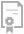Oracle Corporation 2009

ORACLE CERTIFIED ASSOCIATE

## Address

Sector-60 Mohali, Chandigarh, India - 160059

## Verified Info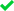ID VerifiedEducation VerifiedPhone VerifiedEmail VerifiedFacebook Verified

Report this Profile

Is this listing inaccurate or duplicate? Any other problem?

Please tell us about the problem and we will fix it.

Please describe the problem that you see in this page.

Type the letters as shown below *

Please enter the letters as show below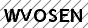Nursery-KG Tuition

Class Location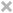Online (video chat via skype, google hangout etc)Student's HomeTutor's Home

Years of Experience in Nursery-KG Tuition

2

Subject

English, Drawing, Mathematics, EVS

Taught in School or College

No

Teaching Experience in detail in Nursery-KG Tuition

Teaching all subjects from Nur -KG students recently at my home based centre.

## Reviews (8)

this is test message this is test message this is test message this is test message this is test message this is test message this is test message

5.0 out of 5.0 8 reviews

Mandeep Kaur https://s3-ap-southeast-1.amazonaws.com/tv-prod/member/photo/5866164-small.jpg Sector-60 Mohali
5.0058
Mandeep Kaur
H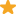"Her teaching methodology is very good. It helps children to understand very well. She is an organized professional with proven teaching, guidance, and counseling skills. She resolve problems and conflicts professionally. Have the ability to communicate complex information in a simple and entertaining manner. "

Reply by Mandeep

thank u himani

Mandeep Kaur
A"I am fully satisfied with Mandeep Mam's way of teaching kids.The way she handle kids is so nice that kids never want to miss her classes anyways. My daughter is in KG and she love to go to her classes.I surely recommend her. "

Reply by Mandeep

thankyou very much

Mandeep Kaur
M"I am fully satisfied with mandeep mam's way of teaching kids.The way she handle kids is so nice. That kids never want to miss her classes anyways. I surely recommend her."

Reply by Mandeep

thank u very muchh

Mandeep Kaur
S"Very much well satisfied experience with mandeep mam's teaching.She is teaching my son who is in 5th grade mathematics and he is also engaged in her cuemath program. He is very much satisfied with her way of teaching. "

Reply by Mandeep

thank you very much

Have you attended any class with Mandeep? Write a Review

## FAQs

1. Which Nursery-KG subjects do you tutor for?

English, Drawing, Mathematics and others

2. Do you have any prior teaching experience?

No

3. Which classes do you teach?

I teach Class 6 Tuition, Class 7 Tuition, Class I-V Tuition and Nursery-KG Tuition Classes.

4. Do you provide a demo class?

Yes, I provide a free demo class.

5. How many years of experience do you have?

I have been teaching for 2 years.

## Answers by Mandeep (8)

Answered on 19/06/2019 CBSE/Class 10/Mathematics/UNIT II: Algebra/Quadratic Equations/NCERT Solutions/Exercise 4.4

For a quadratic equation ax² + bx + c =0, the term b² - 4ac is called discriminant (D) of the quadratic equation because it determines whether the quadratic equation has real roots or not ( nature of roots).D= b² - 4acSo a quadratic equation ax² + bx + c =0, hasi) Two... ...more

For a quadratic equation ax² + bx + c =0,
the term b² - 4ac is called discriminant (D) of
the quadratic equation
because it  determines  whether the quadratic equation has real roots or not ( nature of roots).

D=  b² - 4ac

So a quadratic equation ax² + bx + c =0, has

i) Two distinct real roots, if b² - 4ac >0 , then x= -b/2a + √D/2a  &x= -b/2a - √D/2a

ii) Two equal real roots, if b² - 4ac = 0 , then x= -b/2a or -b/2a

iii) No real roots, if b² - 4ac <0

Solution:

i)

x² – 3x + 5 = 0

Comparing it with ax² + bx + c = 0, we get

a = 2, b = -3 and c = 5

Discriminant (D) = b² – 4ac

⇒ ( – 3)2 – 4 (2) (5) = 9 – 40
⇒ – 31<0

As b2 – 4ac < 0,

Hence, no real root is possible .

(ii) 3x² – 4√3x + 4 = 0

Comparing it with ax² + bx + c = 0, we get

a = 3, b = -4√3 and c = 4

Discriminant(D) = b² – 4ac

⇒ (-4√3)2 – 4(3)(4)

⇒ 48 – 48 = 0

As b² – 4ac = 0,
Hence,  real roots exist & they are equal to each other.

the roots will be –b/2a and –b/2a.

-b/2a = -(-4√3)/2×3 = 4√3/6 = 2√3/3

multiplying the numerator & denominator by √3

(2√3) (√3) / (3)(√3)  = 2 ×3 / 3 ×√3 = 2/√3

Hence , the equal roots are 2/√3 and 2/√3.

(iii) 2x² – 6x + 3 = 0

Comparing this equation with ax² + bx + c = 0, we get

a = 2, b = -6, c = 3

Discriminant (D)= b² – 4ac

= (-6)2 – 4 (2) (3)
= 36 – 24 = 12
As b2 – 4ac > 0,
Hence, two distinct real roots exist for this equation

x= -b/2a + √D/2a  &

x= -b/2a - √D/2a

x= (6+√12) / 2×2= 6+√4×3 /4  = 6 + 2√3 /4  = 2( 3 + √3) 4  = 3 + √3 /2

x = 3 + √3 /2

x= (6-√12) / 2×2= 6-√4×3 /4  = 6 - 2√3 /4  = 2( 3 - √3) 4  = 3 - √3 /2

x= 3 - √3 /2

Hence the real roots are 3 + √3 /2   & 3 -√3 /2
Answers 5 Comments
Dislike Bookmark

Answered on 19/06/2019 CBSE/Class 10/Mathematics/UNIT II: Algebra/Quadratic Equations/NCERT Solutions/Exercise 4.3

Let the sides of first and second square be a and b. Area of first square = (a)² And, Area of second square = (b)²According to question,(a)² + (b)² = 468 m² ------------(1).Perimeter of first square = 4 × aand,Perimeter of second square = 4 × bAccording... ...more

Let the sides of first and second square be a and b.

Area of first square = (a)²

And,

Area of second square = (b)²

According to question,

(a)² + (b)² = 468 m² ------------(1).

Perimeter of first square = 4 × a

and,

Perimeter of second square = 4 × b

According to question,

4a - 4b = 24 -----------(2)

From equation (2) we get,

4a - 4b = 24

4(a-b) = 24

a - b = 24/4

a - b = 6

a = 6+b ---------(3)

Putting the value of a in equation (1)

(a)² + (b)² = 468

(6+b)² + (b)² = 468

(6)² + (b)² + 2 × 6 × b + (b)² = 468

36 + b² + 12b + b² = 468

2b² + 12b - 468 +36 = 0

2b² + 12b -432 = 0

2( b² + 6b - 216) = 0

b² + 6b - 216 = 0

b² + 18b - 12b -216 = 0

b(b+18) - 12(b+18) = 0

(b+18) (b-12) = 0

(b+18) = 0 Or (b-12) = 0

b = -18 OR b = 12

Putting b = 12 in EQUATION (3)

a = 6+b = 6+12 = 18

Side of first square = a = 18 m

and,

Side of second square = b = 12 m
Answers 14 Comments
Dislike Bookmark

Answered on 19/06/2019 CBSE/Class 10/Mathematics/UNIT II: Algebra/Quadratic Equations/NCERT Solutions/Exercise 4.3

Train travels = 132/x hrThe time taken by the express train = {(132)/x+11} hrAccording to the question{132/(x+11)} = 132/x + 1After taking L.C.M. of 132/x +1 and then solving it we get (132+x)/x.Now,{132/(x+11)} = (132+x)/xBy cross multiplying, we get132x = x²+132x+11x+1452x² + 11x - 1452 =... ...more

Train travels = 132/x hr
The time taken by the express train = {(132)/x+11} hr
According to the question
{132/(x+11)} = 132/x + 1
After taking L.C.M. of 132/x +1 and then solving it we get (132+x)/x.
Now,
{132/(x+11)} = (132+x)/x
By cross multiplying, we get
132x = x²+132x+11x+1452
x² + 11x - 1452 = 0
x² + 44x - 33x - 1452 = 0
x(x+44) - 33(x+44) = 0
(x-33) (x+44) = 0
x = -44 or x = 33
As the speed cannot be in negative

therefore, x = 33

or

the speed of the passenger train = 33 km/hr and the speed is 33 + 11 = 44 km/hr

Answers 5 Comments
Dislike Bookmark

Answered on 19/06/2019 CBSE/Class 10/Mathematics/UNIT II: Algebra/Quadratic Equations/NCERT Solutions/Exercise 4.3

Let the time taken by the smaller diameter tap = x larger = x-10 total time taken = 75 /8 portion filled in one hour by smaller diameter tap = 1/x and by larger diamter tap = 1/x-10 1/x + 1/x-10 = 8/75 x-10+x/x(x-10) = 8/75 2x+10/x²-10x = 8/75 8(x²-10x) = 75 ×2 (x-5) 8/2 (x²-10x)... ...more

Let the time taken by the smaller diameter tap = x

larger = x-10

total time taken = 75 /8

portion filled in one hour by smaller diameter tap = 1/x

and by larger diamter tap = 1/x-10

1/x + 1/x-10 = 8/75

x-10+x/x(x-10) = 8/75

2x+10/x²-10x = 8/75

8(x²-10x) = 75 ×2 (x-5)

8/2 (x²-10x) = 75 (x-5)

4x²-40x = 75x-375

4x² -40x-75x +375 = 0

4x²-115x + 375 = 0

4x²-100x-15x +375 = 0

4x(x-25)-15(x-25)=0

(x-25)(4x-15)

x= 25

x= 15/4

If x= 25

the x-10 = 25-10 = 15

if x = 15/4

x-10 = 15/4 - 10 = 15-40/4 = -25/4

Since ,

Time cannot be negative

Therefore x = 25

Answers 7 Comments
Dislike Bookmark

Answered on 19/06/2019 CBSE/Class 10/Mathematics/UNIT II: Algebra/Quadratic Equations/NCERT Solutions/Exercise 4.3

Let shorter side be x Diagonal = x+60 Longer side =x+30 By applying Pythagoras theorem Shorter side = 90m Longer side =120m
Answers 17 Comments
Dislike Bookmark
Nursery-KG Tuition 5.0

Class LocationOnline (video chat via skype, google hangout etc)Student's HomeTutor's Home

Years of Experience in Nursery-KG Tuition

2

Subject

English, Drawing, Mathematics, EVS

Taught in School or College

No

Teaching Experience in detail in Nursery-KG Tuition

Teaching all subjects from Nur -KG students recently at my home based centre.

Class I-V Tuition 5.0

Class LocationOnline (video chat via skype, google hangout etc)Student's HomeTutor's Home

Years of Experience in Class I-V Tuition

2

Fees

₹ 1200 per hour

Board

State, ICSE, CBSE

CBSE Subjects taught

Mathematics, English, Science, Social Science, Hindi, Computers, EVS

ICSE Subjects taught

Computer science, Science, English, EVS, Hindi, Social Studies, Mathematics

Taught in School or College

No

State Syllabus Subjects taught

English, Mathematics, Science, Social Science, Computer Science, Hindi, EVS

Class 6 Tuition 5.0

Class LocationOnline (video chat via skype, google hangout etc)Student's HomeTutor's Home

Years of Experience in Class 6 Tuition

2

Board

CBSE, ICSE

CBSE Subjects taught

Punjabi, Computers, English, Mathematics, Science, Hindi, EVS, Social Science

ICSE Subjects taught

Punjabi, English, Hindi, EVS, Physics, Mathematics, Computer Science

Taught in School or College

No

Class 7 Tuition 5.0

Class LocationOnline (video chat via skype, google hangout etc)Student's HomeTutor's Home

Years of Experience in Class 7 Tuition

3

Board

CBSE, ICSE, State

CBSE Subjects taught

Punjabi, Computers, English, Mathematics, Science, Hindi, EVS, Social Science

ICSE Subjects taught

History, English, Geography, Hindi, EVS, Mathematics, Computer Science

Taught in School or College

No

State Syllabus Subjects taught

EVS, Hindi, Punjabi, Social science, Mathematics, English, Science

## Answers by Mandeep (8)

Answered on 19/06/2019 CBSE/Class 10/Mathematics/UNIT II: Algebra/Quadratic Equations/NCERT Solutions/Exercise 4.4

For a quadratic equation ax² + bx + c =0, the term b² - 4ac is called discriminant (D) of the quadratic equation because it determines whether the quadratic equation has real roots or not ( nature of roots).D= b² - 4acSo a quadratic equation ax² + bx + c =0, hasi) Two... ...more

For a quadratic equation ax² + bx + c =0,
the term b² - 4ac is called discriminant (D) of
the quadratic equation
because it  determines  whether the quadratic equation has real roots or not ( nature of roots).

D=  b² - 4ac

So a quadratic equation ax² + bx + c =0, has

i) Two distinct real roots, if b² - 4ac >0 , then x= -b/2a + √D/2a  &x= -b/2a - √D/2a

ii) Two equal real roots, if b² - 4ac = 0 , then x= -b/2a or -b/2a

iii) No real roots, if b² - 4ac <0

Solution:

i)

x² – 3x + 5 = 0

Comparing it with ax² + bx + c = 0, we get

a = 2, b = -3 and c = 5

Discriminant (D) = b² – 4ac

⇒ ( – 3)2 – 4 (2) (5) = 9 – 40
⇒ – 31<0

As b2 – 4ac < 0,

Hence, no real root is possible .

(ii) 3x² – 4√3x + 4 = 0

Comparing it with ax² + bx + c = 0, we get

a = 3, b = -4√3 and c = 4

Discriminant(D) = b² – 4ac

⇒ (-4√3)2 – 4(3)(4)

⇒ 48 – 48 = 0

As b² – 4ac = 0,
Hence,  real roots exist & they are equal to each other.

the roots will be –b/2a and –b/2a.

-b/2a = -(-4√3)/2×3 = 4√3/6 = 2√3/3

multiplying the numerator & denominator by √3

(2√3) (√3) / (3)(√3)  = 2 ×3 / 3 ×√3 = 2/√3

Hence , the equal roots are 2/√3 and 2/√3.

(iii) 2x² – 6x + 3 = 0

Comparing this equation with ax² + bx + c = 0, we get

a = 2, b = -6, c = 3

Discriminant (D)= b² – 4ac

= (-6)2 – 4 (2) (3)
= 36 – 24 = 12
As b2 – 4ac > 0,
Hence, two distinct real roots exist for this equation

x= -b/2a + √D/2a  &

x= -b/2a - √D/2a

x= (6+√12) / 2×2= 6+√4×3 /4  = 6 + 2√3 /4  = 2( 3 + √3) 4  = 3 + √3 /2

x = 3 + √3 /2

x= (6-√12) / 2×2= 6-√4×3 /4  = 6 - 2√3 /4  = 2( 3 - √3) 4  = 3 - √3 /2

x= 3 - √3 /2

Hence the real roots are 3 + √3 /2   & 3 -√3 /2
Answers 5 Comments
Dislike Bookmark

Answered on 19/06/2019 CBSE/Class 10/Mathematics/UNIT II: Algebra/Quadratic Equations/NCERT Solutions/Exercise 4.3

Let the sides of first and second square be a and b. Area of first square = (a)² And, Area of second square = (b)²According to question,(a)² + (b)² = 468 m² ------------(1).Perimeter of first square = 4 × aand,Perimeter of second square = 4 × bAccording... ...more

Let the sides of first and second square be a and b.

Area of first square = (a)²

And,

Area of second square = (b)²

According to question,

(a)² + (b)² = 468 m² ------------(1).

Perimeter of first square = 4 × a

and,

Perimeter of second square = 4 × b

According to question,

4a - 4b = 24 -----------(2)

From equation (2) we get,

4a - 4b = 24

4(a-b) = 24

a - b = 24/4

a - b = 6

a = 6+b ---------(3)

Putting the value of a in equation (1)

(a)² + (b)² = 468

(6+b)² + (b)² = 468

(6)² + (b)² + 2 × 6 × b + (b)² = 468

36 + b² + 12b + b² = 468

2b² + 12b - 468 +36 = 0

2b² + 12b -432 = 0

2( b² + 6b - 216) = 0

b² + 6b - 216 = 0

b² + 18b - 12b -216 = 0

b(b+18) - 12(b+18) = 0

(b+18) (b-12) = 0

(b+18) = 0 Or (b-12) = 0

b = -18 OR b = 12

Putting b = 12 in EQUATION (3)

a = 6+b = 6+12 = 18

Side of first square = a = 18 m

and,

Side of second square = b = 12 m
Answers 14 Comments
Dislike Bookmark

Answered on 19/06/2019 CBSE/Class 10/Mathematics/UNIT II: Algebra/Quadratic Equations/NCERT Solutions/Exercise 4.3

Train travels = 132/x hrThe time taken by the express train = {(132)/x+11} hrAccording to the question{132/(x+11)} = 132/x + 1After taking L.C.M. of 132/x +1 and then solving it we get (132+x)/x.Now,{132/(x+11)} = (132+x)/xBy cross multiplying, we get132x = x²+132x+11x+1452x² + 11x - 1452 =... ...more

Train travels = 132/x hr
The time taken by the express train = {(132)/x+11} hr
According to the question
{132/(x+11)} = 132/x + 1
After taking L.C.M. of 132/x +1 and then solving it we get (132+x)/x.
Now,
{132/(x+11)} = (132+x)/x
By cross multiplying, we get
132x = x²+132x+11x+1452
x² + 11x - 1452 = 0
x² + 44x - 33x - 1452 = 0
x(x+44) - 33(x+44) = 0
(x-33) (x+44) = 0
x = -44 or x = 33
As the speed cannot be in negative

therefore, x = 33

or

the speed of the passenger train = 33 km/hr and the speed is 33 + 11 = 44 km/hr

Answers 5 Comments
Dislike Bookmark

Answered on 19/06/2019 CBSE/Class 10/Mathematics/UNIT II: Algebra/Quadratic Equations/NCERT Solutions/Exercise 4.3

Let the time taken by the smaller diameter tap = x larger = x-10 total time taken = 75 /8 portion filled in one hour by smaller diameter tap = 1/x and by larger diamter tap = 1/x-10 1/x + 1/x-10 = 8/75 x-10+x/x(x-10) = 8/75 2x+10/x²-10x = 8/75 8(x²-10x) = 75 ×2 (x-5) 8/2 (x²-10x)... ...more

Let the time taken by the smaller diameter tap = x

larger = x-10

total time taken = 75 /8

portion filled in one hour by smaller diameter tap = 1/x

and by larger diamter tap = 1/x-10

1/x + 1/x-10 = 8/75

x-10+x/x(x-10) = 8/75

2x+10/x²-10x = 8/75

8(x²-10x) = 75 ×2 (x-5)

8/2 (x²-10x) = 75 (x-5)

4x²-40x = 75x-375

4x² -40x-75x +375 = 0

4x²-115x + 375 = 0

4x²-100x-15x +375 = 0

4x(x-25)-15(x-25)=0

(x-25)(4x-15)

x= 25

x= 15/4

If x= 25

the x-10 = 25-10 = 15

if x = 15/4

x-10 = 15/4 - 10 = 15-40/4 = -25/4

Since ,

Time cannot be negative

Therefore x = 25

Answers 7 Comments
Dislike Bookmark

Answered on 19/06/2019 CBSE/Class 10/Mathematics/UNIT II: Algebra/Quadratic Equations/NCERT Solutions/Exercise 4.3

Let shorter side be x Diagonal = x+60 Longer side =x+30 By applying Pythagoras theorem Shorter side = 90m Longer side =120m
Answers 17 Comments
Dislike Bookmark
Load More

Mandeep K. describes herself as Academic Tutor & Cuemath Teacher. She conducts classes in Class 6 Tuition, Class 7 Tuition and Class I-V Tuition. Mandeep is located in Sector-60 Mohali, Chandigarh. Mandeep takes Regular Classes- at her Home. She has 3 years of teaching experience . Mandeep has completed Bachelor of Computer Science (B.Sc. (Computer Science)) from Kurukshetra University in 2005 and Master of Computer Applications (M.C.A.) from Kurukshetra University, Kurukshetra in 2009. She is well versed in Hindi, English and Punjabi. Mandeep has got 8 reviews till now with 100% positive feedback.

•• Want to learn from Mandeep?

• Contact Now
X

Share this Profile

Recommended Profiles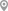Sector-47, ChandigarhSector-30 C, ChandigarhSector-49, ChandigarhSector-60 Mohali, ChandigarhSector-14, ChandigarhSector-15, Chandigarh

X

Post your Learning Need

Let us shortlist and give the best tutors and institutes.

or

Send Enquiry to Mandeep

Let Mandeep know you are interested in their class

Reply to 's review

Enter your reply*

1500/1500

Please enter your reply

Your reply should contain a minimum of 10 charactersYour reply has been successfully submitted.

UrbanPro.com is India's largest network of most trusted tutors and institutes. Over 55 lakh students rely on UrbanPro.com, to fulfill their learning requirements across 1,000+ categories. Using UrbanPro.com, parents, and students can compare multiple Tutors and Institutes and choose the one that best suits their requirements. More than 7.5 lakh verified Tutors and Institutes are helping millions of students every day and growing their tutoring business on UrbanPro.com. Whether you are looking for a tutor to learn mathematics, a German language trainer to brush up your German language skills or an institute to upgrade your IT skills, we have got the best selection of Tutors and Training Institutes for you. Read more